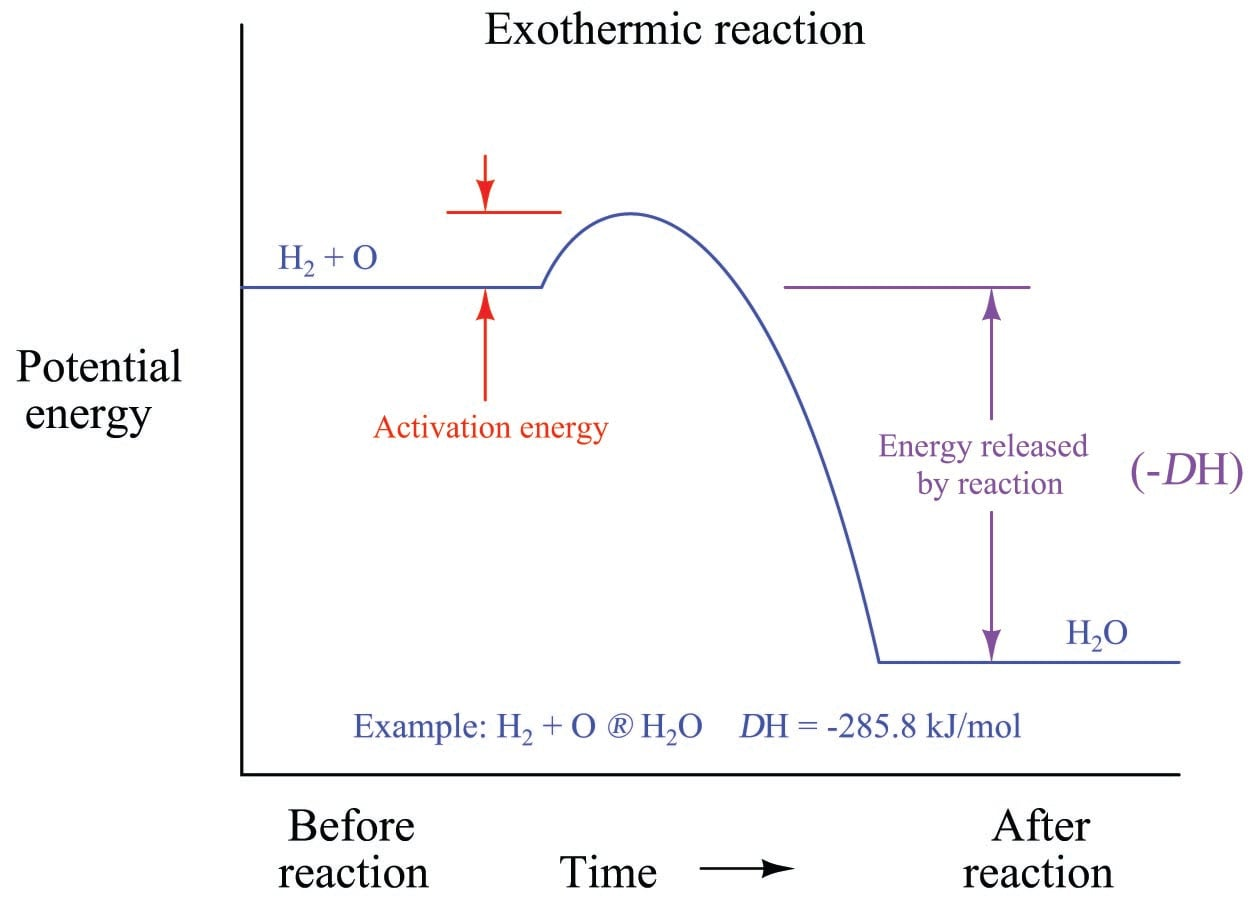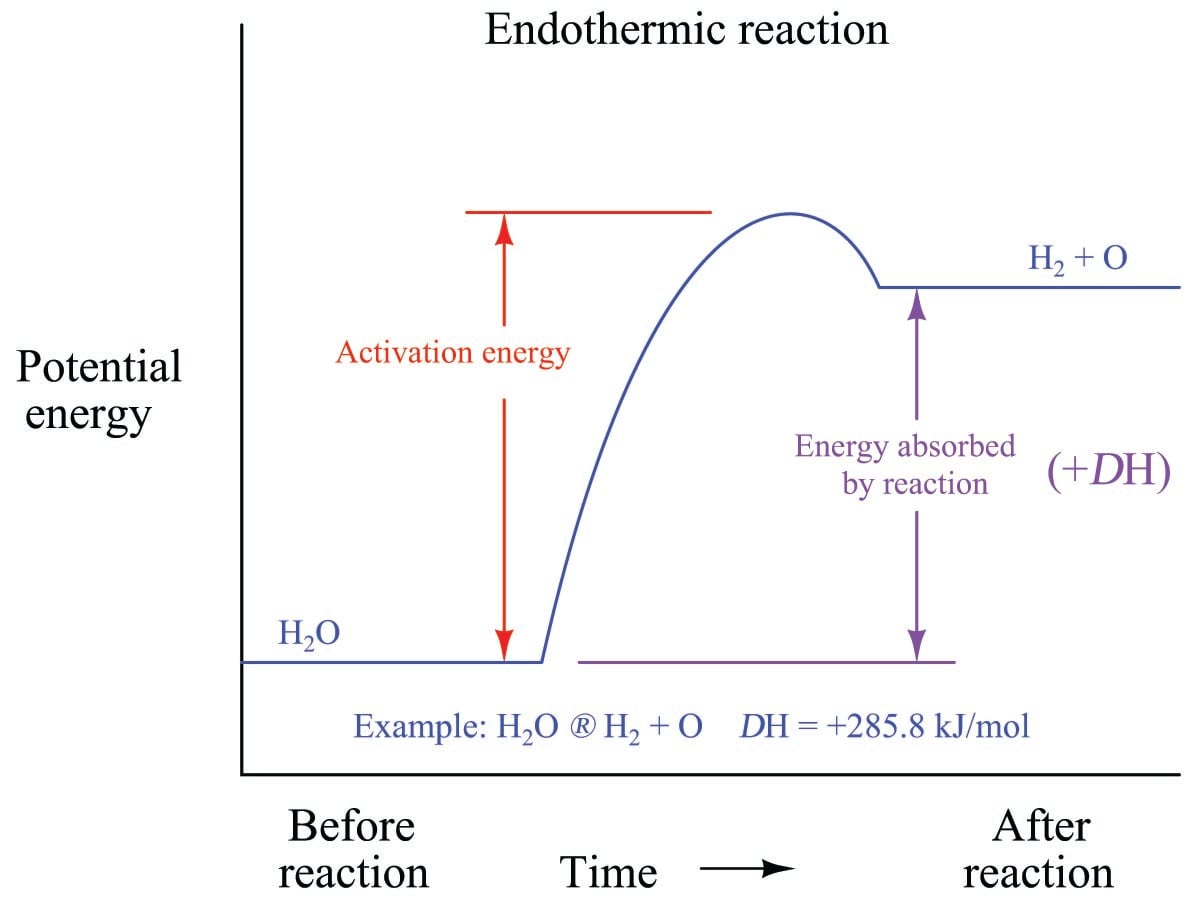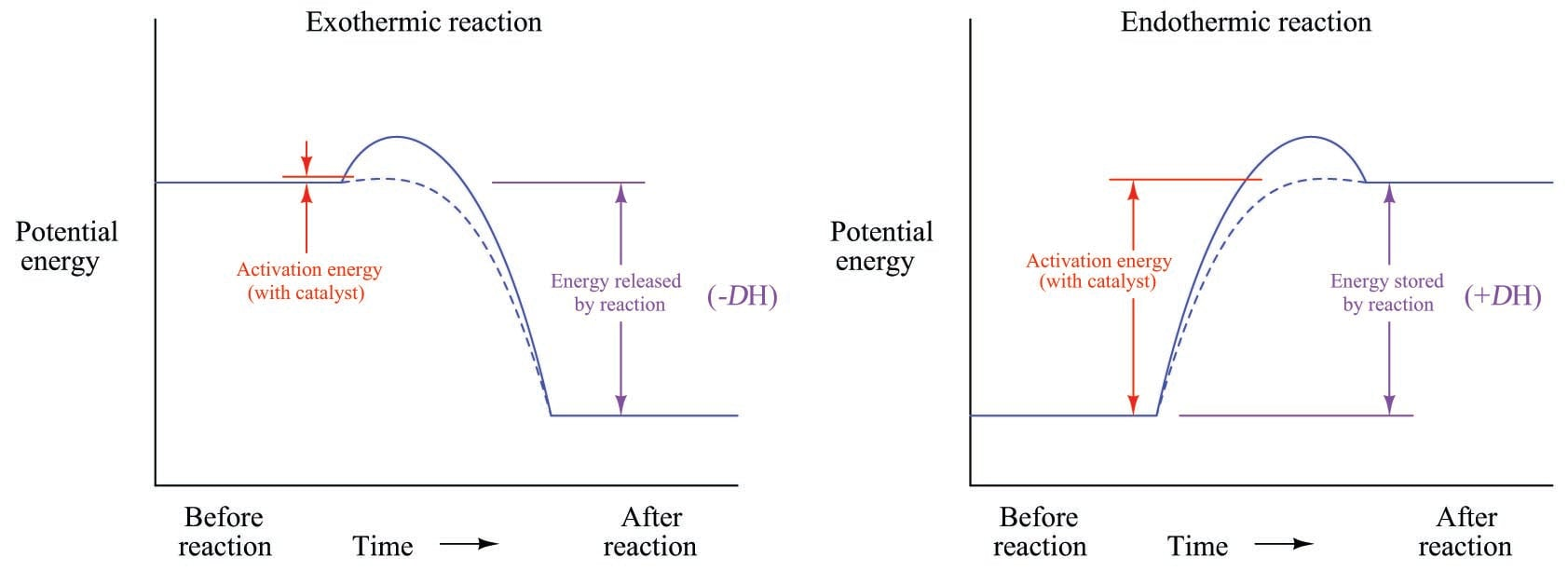Energy in Chemical Reactions

Chapter 3 - Chemistry in Industrial Instrumentation

A chemical reaction resulting in a net release of energy is called exothermic. Conversely, a chemical reaction requiring a net input of energy to occur is called endothermic. The relationship between chemical reactions and energy exchange corresponds to the breaking or making of chemical bonds. Atoms bonded together represent a lower state of total energy than those same atoms existing separately, all other factors being equal. Thus, when separate atoms join together to form a molecule, they go from a high state of energy to a low state of energy, releasing the difference in energy in some form (heat, light, etc.). Conversely, an input of energy is required to break that chemical bond and force the atoms to separate.

Chemical bonds are considered “strong” if a large input of energy is required to break them. “Weak” chemical bonds, by contrast, only require modest inputs of energy to disrupt. Thus, the strength of a bond is inversely proportional to the energy state of the molecule: atoms falling into very low energy states when joining together to form a molecule enjoy a strong bond because a large investment of energy is required to raise those atoms’ energy states high enough to sever their bond.

An example of a strong bond is that which exists between two atoms of hydrogen (H) and one atom of oxygen (O) when forming water (H$$_{2}$$O). When hydrogen and oxygen atoms bond together to form water, they release energy. This, by definition, is an exothermic reaction, but we know it better as combustion: hydrogen is flammable in the presence of oxygen. A reversal of this reaction occurs when water is subjected to an electrical current, breaking water molecules up into hydrogen and oxygen gas molecules. This process of forced separation requires a substantial input of energy to accomplish, which by definition makes it an endothermic reaction. Specifically, the use of electricity to cause a chemical reaction is called electrolysis.

An even stronger bond is that formed between aluminum (Al) and oxygen (O) to make alumina (Al$$_{2}$$O$$_{3}$$), a ceramic powder at room temperature. The energy state of this molecule is so low that the aluminum-oxygen bonds resist dissolution even at extremely high temperatures, explaining the high melting point and relative non-reactivity of this substance.

Heats of reaction and activation energy

The amount of energy exchanged (either absorbed or released) in a chemical reaction is often expressed as a numerical quantity to the right of the equation, labeled $$\Delta H$$, usually defined at a reference temperature of 298 Kelvin (25 degrees Celsius). A negative $$\Delta H$$ value signifies an exothermic (heat-releasing) reaction, while a positive $$\Delta H$$ value signifies an endothermic (heat-absorbing) reaction. The combustion of hydrogen and oxygen to form liquid water is an example of the former, and the electrolysis of water to yield hydrogen and oxygen gas is an example of the latter:

$\hbox{H}_2 + \hbox{O} \rightarrow \hbox{H}_2\hbox{O} \hskip 30pt \Delta H_{298} = -285.8 \hbox{ kJ mol}^{-1} \hskip 10pt \hbox{\textit{(exothermic)}}$

$\hbox{H}_2\hbox{O} \rightarrow \hbox{H}_2 + \hbox{O} \hskip 30pt \Delta H_{298} = +285.8 \hbox{ kJ mol}^{-1} \hskip 10pt \hbox{\textit{(endothermic)}}$

This energy value, commonly referred to as the heat of reaction or enthalpy of reaction, is expressed per mole of the reactants and products shown. The “$$-1$$” exponent applied to “mole” is simply a fancy way of saying “per mole”, as an alternative to using a fraction bar.

While the mathematical sign of $$\Delta H$$ may seem confusing at first (“Why is it negative when energy is released?”), it makes sense from the perspective of energy states before and after the reaction. In an exothermic (heat-releasing) reaction, the products are left at a lower state of energy than the reactants began with, and a negative value of $$\Delta H$$ signifies this. The sign of $$\Delta H$$, then, is an expression of the change in energy state from reactants (input) to products (output), not an expression of the energy liberated from the reaction. Even though the term $$\Delta H$$ is called the “heat of reaction” it really refers to the change in potential energy of the matter as a consequence of the chemical reaction.

The fact that hydrogen and oxygen as separate gases possess potential energy does not mean they are guaranteed to spontaneously combust when brought together. By analogy, just because rocks sitting on a hillside possess potential energy (by virtue of being elevated above the hill’s base) does not means all rocks in the world spontaneously roll downhill. Some rocks need a push to get started because they are caught on a ledge or resting in a depression on the hillside. Likewise, many exothermic reactions require an initial investment of energy before they can proceed. In the case of hydrogen and oxygen, what is generally needed is a spark to initiate the reaction. This initial requirement of input energy is called the activation energy of the reaction.

Activation energy may be shown in graphical form. For an exothermic reaction, it appears as a “hill” that must be climbed before the total energy can fall to a lower (than original) level:For an endothermic reaction, activation energy is much greater, a part of which never returns but is stored in the reaction products as potential energy:A catalyst is a substance that works to minimize activation energy in a chemical reaction without being altered by the reaction itself. Catalysts are popularly used in industry to accelerate both exothermic and endothermic reactions, reducing the gross amount of energy that must be initially input to a process to make a reaction occur. A common example of a catalyst is the catalytic converter installed in the exhaust pipe of an automobile engine, helping to oxidize unburnt fuel molecules and certain combustion products such as carbon monoxide (CO) to compounds which are not as polluting. Without a catalytic converter, the exhaust gas temperature is not hot enough to overcome the activation energy of these reactions, and so they will not occur (at least not at the rate necessary to make a significant difference). The presence of the catalyst allows the reactions to progress quickly at typical engine exhaust temperatures.

The effect of a catalyst on activation energy may be shown by the following graphs, the dashed-line curve showing the energy progression with a catalyst and the solid-line curve showing the reaction progressing without the benefit of a catalyst:It should be noted that the presence of a catalyst has absolutely no effect on the net energy loss or gain resulting from a chemical reaction. That is to say, the heat of reaction ($$\Delta H$$) stands independent of catalytic assistance: with or without a catalyst, the difference in potential energy before and after a reaction will be the same. The only difference a catalyst makes to a chemical reaction is how much energy must be initially invested to spark the reaction. To use the example of hydrogen and oxygen gas once again, the presence of a catalyst does not cause the combustion of hydrogen and oxygen to release more energy. All the catalyst does is make it easier for the combustion to begin.

Heats of formation and Hess’s Law

As we have seen, the formation of new chemical bonds between atoms is an energy-releasing process (i.e. exothermic), while the dissolution of chemical bonds is an energy-absorbing process (i.e. endothermic). Given the fact that the Law of Energy Conservation is universal, it stands to reason we ought to be able to mathematically balance the potential energy held by reactants, the potential energy held by the products, and the amount of energy either released or absorbed by the reaction.

Let us begin our exploration of this concept with the formation of carbon dioxide (CO$$_{2}$$) from the combustion of elemental carbon (C) and oxygen molecules (O$$_{2}$$):

$\hbox{C} + \hbox{O}_2 \to \hbox{CO}_2 \hskip 30pt \Delta H = -393.5 \hbox{ kJ mol}^{-1}$

As we can see, this reaction is exothermic: the products have a lower energy than the reactants, losing 393.5 kilojoules of energy for every mole of carbon dioxide formed by this reaction.

If we are to account for all the energy entering and exiting a chemical reaction, we must have some means of quantifying the amount of energy stored within both the reactants and the products as well as the amount of energy released or absorbed by the reaction itself. Quantifying the amount of chemical potential possessed by atoms and molecules is difficult if not impossible to do in any absolute sense, and so the common practice is to arbitrarily assign an energy value of zero to chemical elements in their normal states at standard temperature and pressure (293.15 Kelvin and 1 atmosphere, abbreviated “STP”). This point of reference will be the norm for any subsequent determinations of chemical potential energy. The standard heat of formation or standard enthalpy of formation ($$\Delta H_f^{\circ}$$, or sometimes $$\Delta_f H^{\circ}$$) for any substance is thus defined as the amount of energy gained or lost when one mole of that substance is formed from its constituent elements at STP. A superscripted “$$\circ$$” symbol represents conditions of standard temperature and pressure.

We know that the phase of a substance (i.e. solid, liquid, gas) affects how much energy it contains, and therefore in order to accurately account for all energy we must represent the phase of each substance when we specify heats of formation. Since the natural state of carbon is solid (s) at STP while the natural state of oxygen is gas (g) at STP, we will represent those states as letters within parentheses when defining their heats of formation:

$\Delta H_f^{\circ} \hbox{ (C, s)} = 0 \hbox{ kJ mol}^{-1} \hskip 30pt \hbox{Heat of formation for solid carbon at STP}$

$\Delta H_f^{\circ} \hbox{ (O}_2 \hbox{, g)} = 0 \hbox{ kJ mol}^{-1} \hskip 14pt \hbox{Heat of formation for gaseous oxygen at STP}$

It takes no gain or loss of energy at all to form solid carbon (C) or gaseous oxygen molecules (O$$_{2}$$) at STP because those elements are already in those forms at STP. The only way we will ever have a non-zero $$\Delta H_f^{\circ}$$ value is if the substance in question is a compound (i.e. comprised of multiple elements joined by chemical bonds) or if the substance in question is an element in some unusual energy state (e.g. ionization).

Since we already know the combustion of one atom of carbon with one molecule of oxygen liberates 393.5 kilojoules of heat energy, we may conclude the heat of formation for carbon dioxide gas (cooled down to the standard temperature of 293.15 Kelvin) must be $$-$$393.5 kJ mol$$^{-1}$$, since this is precisely how much energy is liberated when carbon dioxide is formed from its constituent elements. Representing all these figures in a table helps us make sense of it all:

Reactant Reactant Reaction Product
C(s) O$$_{2}$$(g) $$\to$$ CO$$_{2}$$(g)
$$\Delta H_f^{\circ}$$ = 0 kJ mol$$^{-1}$$ $$\Delta H_f^{\circ}$$ = 0 kJ mol$$^{-1}$$ $$\Delta H^{\circ}$$ = $$-393.5$$ kJ mol$$^{-1}$$ $$\Delta H_f^{\circ}$$ = $$-393.5$$ kJ mol$$^{-1}$$

Stated verbally, the combined heats of formation for all reactants plus the heat of reaction yields the combined heats of formation for all products. Put into simpler terms, the energy contained by the reactants plus the change in energy wrought by the reaction gives us the energy left inside the products. The mathematical formulation of this principle is as follows:

$\Sigma \left[ \Delta H_f^{\circ} \hbox{ (Reactants)} \right] + \Delta H^{\circ} \hbox{ (Reaction)} = \Sigma \left[ \Delta H_f^{\circ} \hbox{ (Products)} \right]$

The practical application of this is that we may calculate the exact amount of heat liberated or absorbed by any chemical reaction, if only we know in advance the heats of formation for all the reactants and products. Fortunately for our reference, chemists have tabulated standard heats of formation for a great many substances.

Hess’s Law states that this accounting of energy is true regardless of the reaction path. For example, if the combustion of carbon with oxygen proceeds in one step (C + O$$_{2}$$ $$\to$$ CO$$_{2}$$), the overall heat of reaction will be precisely the same as for any other series of steps resulting in the same product(s) from the same reactant(s), for example the partial combustion of carbon to form carbon monoxide (C + O $$\to$$ CO) followed by the subsequent combustion of carbon monoxide (CO + O $$\to$$ CO$$_{2}$$). Just as we saw with catalytically-aided chemical reactions, the total heat of reaction is strictly a function of the reactants and the products, not of any process or path by which the reaction may proceed. The Law of Energy Conservation is indeed iron-clad!

Let us investigate a practical application where we employ heats of formation to calculate the heat of a chemical reaction. In this case, we will consider the combustion of propane fuel gas (C$$_{3}$$H$$_{8}$$) in the presence of pure oxygen gas (O$$_{2}$$), producing liquid water (H$$_{2}$$O) and gaseous carbon dioxide (CO$$_{2}$$) as products:

$\hbox{C}_3\hbox{H}_8\hbox{(g)} + 5\hbox{O}_2\hbox{(g)} \to 4\hbox{H}_2\hbox{O}\hbox{(l)} + 3\hbox{CO}_2\hbox{(g)}$

To begin, we must identify the standard heats of formation for each of these substances at STP from a suitable reference:

• Propane gas $$\Delta H_f^{\circ}$$ = $$-$$103.8 kJ mol$$^{-1}$$
• Oxygen gas $$\Delta H_f^{\circ}$$ = 0 kJ mol$$^{-1}$$
• Water $$\Delta H_f^{\circ}$$ = $$-$$285.8 kJ mol$$^{-1}$$
• Carbon dioxide gas $$\Delta H_f^{\circ}$$ = $$-$$393.5 kJ mol$$^{-1}$$

Setting these quantities into a table for ease of organization (all heats of formation given in units of kilojoules per mole):

Reactant Reactant Reaction Product Product
C$$_{3}$$H$$_{8}$$(g) 5O$$_{2}$$(g) $$\to$$ 4H$$_{2}$$O(l) 3CO$$_{2}$$(g)
(1)($$-$$103.8) (5)(0) $$\Delta H^{\circ}$$ (4)($$-$$285.8) (3)($$-$$393.5)

Solving for the unknown heat of reaction ($$\Delta H^{\circ}$$):

$\Sigma \left[ \Delta H_f^{\circ} \hbox{ (Reactants)} \right] + \Delta H^{\circ} \hbox{ (Reaction)} = \Sigma \left[ \Delta H_f^{\circ} \hbox{ (Products)} \right]$

$\left[ (1)(-103.8) + (5)(0) \right] + \Delta H^{\circ} = \left[ (4)(-285.8) + (3)(-393.5) \right]$

$\left[ -103.8 \right] + \Delta H^{\circ} = \left[ -1143.2 + -1180.5 \right]$

$\left[ -103.8 \right] + \Delta H^{\circ} = \left[ -2323.7 \right]$

$\Delta H^{\circ} = -2323.7 + 103.8$

$\Delta H^{\circ} = -2219.9 \hbox{ kJ per mole of propane fuel}$

The large, negative value of $$\Delta H^{\circ}$$ tells us the reaction of propane with oxygen will be highly exothermic.

Published under the terms and conditions of the Creative Commons Attribution 4.0 International Public License